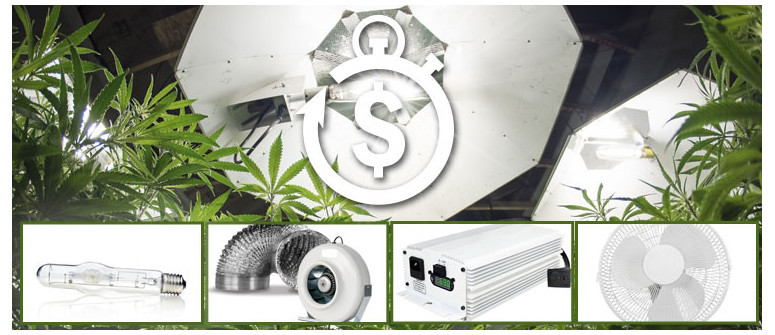# How to calculate the electricity costs for growing cannabis indoorsIf you’re planning an indoor grow, you’ll want to calculate the cost of your electricity consumption to avoid any unwanted surprises. Find out how to calculate the electricity costs for growing weed indoors.

If you’re planning an indoor grow, you’ll want to calculate the cost of your electricity consumption to avoid any unwanted surprises. Below are some rough estimates of electricity costs for growing weed indoors.

Obviously, some growers use bigger equipment, more powerful equipment, including 150, 250, 400, 600 or even 1000 watt lamps. However, a standard 400W lamp (HPS) is considered by many as a pretty good starting point for a basic indoor set up.

To provide a basic example of the costs of a small indoor grow setup, let's imagine we have a small grow tent with one HPS lamp of 400W and the vegetative period takes 4 weeks which is followed by a flowering period of 8 weeks.

You will need several items that require electricity in a basic growtent:

• LAMP. At this moment HPS lamps are still the most used light source in indoor grow setups. For the first 4 weeks, your lamp will be running for about 18 hours a day (given you stick to a regular 18-6 light cycle). Then, during the flowering period, your lamp will be running 12 hours daily for roughly 8 weeks.
• BALLAST. A HPS lamp’s ballast (or current regulator) uses usually around 15% of the entire power of the lamp when it's switched on.
• EXTRACTOR FAN. This item takes care of fresh air by sucking it in from the ventilation holes at the bottom of the tent. In combination with a filter odorless air will exit the tent on top. The extractor fan will be on the entire time (24 hours a day, 7 days a week, for the full 12 weeks). An extractor generally consumes about 30 watts of electricity.
• FAN. A fan is needed for the necessary air movement. Your fan will also be on for the full 12 weeks. Most fans consume about 20 watts of electricity.
Related Article

Electricity costs are generally calculated by kilowatt hours. To calculate this, simply take the total number of watt hours for all of your pieces of equipment and divide this by 1000 (one kilowatt is 1000 watts). This gives you the total of kilowatt hours of electricity your grow room will consume.

Now let's make a calculation:

• Lamp vegetative period = (28 days x 18 hours) x 400W = 201600 watt hours
• Lamp flowering period = (56 days x 12 hours) x 400W = 268800 watt hours
• Ballast: 15% of (201600 + 268800) = 70560 watt hours
• Extractor: (12 weeks: 84 days x 24 hours) x 30 watt: 60480 watt hours.
• Fan: (12 weeks: 84 days x 24 hours) x 20 watt: 40320 watt hours.
• Total watt hours: 641760
• 555.360 watt hours / 1000 = 641.76 kilowatt hours

Now, to work this out in terms of money, simply take the average price of a kilowatt hour in your area (in our case it's 0.21€, or roughly \$0.24 US) and multiply that by the total number of kilowatt hours you calculated earlier.

So, the total electricity costs of running the above indoor grow room is 641.76 x 0.21 = 134.77€ (approx. \$150)

### THINGS TO REMEMBER

Remember that these calculations are made using rough estimates. The exact wattage of your equipment will vary from one manufacturer to another, but these are pretty standard figures. Plus, you may also choose to use different light cycles and keep your plants in their vegetative and flowering phases for more or less time, which will also affect overall costs.

Either way, these figures give you a good baseline of how much a standard personal indoor grow setup costs to run. Plus, it also provides you with the basis to calculate the costs of all your future grows.

Related Article